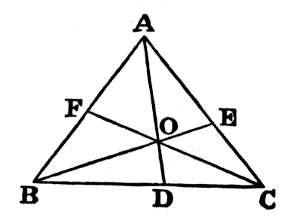#Pillow-Problems: Problem #24

Math Lair Home > Source Material > Pillow-Problems > Problem #24

## Problem:

24.

If, from the vertices of a triangle ABC, the lines AD, BE,CF be drawn, intersecting at O; find the ratio
 DO DA
in terms of the two ratios
 EO EB
,
 FO FC
.
[5/86

24.

 DO DA
+
 EO EB
+
 FO FC
= 1; whence any one can be found in terms of the other two.

## Solution:

24.Because
 DO OA
= △
 △DOC OAC
= △
 △DOB OAB
= △
 △OBC OCA + △OAB
; ∴
 DO DA
= △
 △OBC ABC
.

Similarly,
 EO EB
= △
 △OCA ABC
, and
 FO FC
= △
 △OAB ABC
.

Hence
 DO DA
+
 EO EB
+
 FO FC
= 1

Q.E.F.